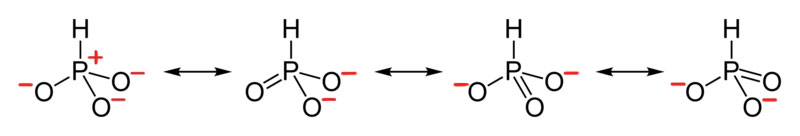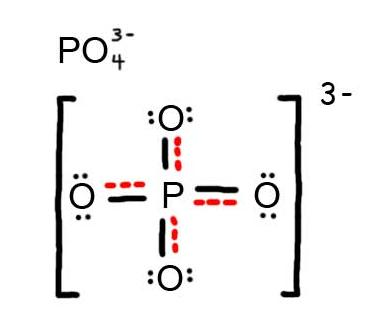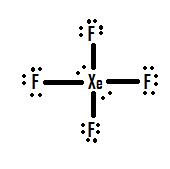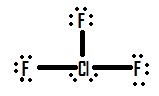# 8.E: Basic Concepts of Chemical Bonding (Exercises)

These are homework exercises to accompany the Textmap created for "Chemistry: The Central Science" by Brown et al. Complementary General Chemistry question banks can be found for other Textmaps and can be accessed here. In addition to these publicly available questions, access to private problems bank for use in exams and homework is available to faculty only on an individual basis; please contact Delmar Larsen for an account with access permission.

## 8.1: CHEMICAL BONDS, LEWIS SYMBOLS AND THE OCTET RULE

### Conceptual Problems

1. The Lewis electron system is a simplified approach for understanding bonding in covalent and ionic compounds. Why do chemists still find it useful?
2. Is a Lewis dot symbol an exact representation of the valence electrons in an atom or ion? Explain your answer.
3. How can the Lewis electron dot system help to predict the stoichiometry of a compound and its chemical and physical properties?
4. How is a Lewis dot symbol consistent with the quantum mechanical model of the atom? How is it different?

3. Lewis dot symbols allow us to predict the number of bonds atoms will form, and therefore the stoichiometry of a compound. The Lewis structure of a compound also indicates the presence or absence of lone pairs of electrons, which provides information on the compound’s chemical reactivity and physical properties.

## 8.2: IONIC BONDING

### Conceptual Problems

1. Describe the differences in behavior between NaOH and CH3OH in aqueous solution. Which solution would be a better conductor of electricity? Explain your reasoning.

2. What is the relationship between the strength of the electrostatic attraction between oppositely charged ions and the distance between the ions? How does the strength of the electrostatic interactions change as the size of the ions increases?

3. Which will result in the release of more energy: the interaction of a gaseous sodium ion with a gaseous oxide ion or the interaction of a gaseous sodium ion with a gaseous bromide ion? Why?

4. Which will result in the release of more energy: the interaction of a gaseous chloride ion with a gaseous sodium ion or a gaseous potassium ion? Explain your answer.

5. What are the predominant interactions when oppositely charged ions are

1. far apart?
2. at internuclear distances close to r0?
3. very close together (at a distance that is less than the sum of the ionic radii)?
6. Several factors contribute to the stability of ionic compounds. Describe one type of interaction that destabilizes ionic compounds. Describe the interactions that stabilize ionic compounds.

7. What is the relationship between the electrostatic attractive energy between charged particles and the distance between the particles?

1. The interaction of a sodium ion and an oxide ion. The electrostatic attraction energy between ions of opposite charge is directly proportional to the charge on each ion (Q1 and Q2 in Equation 9.1). Thus, more energy is released as the charge on the ions increases (assuming the internuclear distance does not increase substantially). A sodium ion has a +1 charge; an oxide ion, a −2 charge; and a bromide ion, a −1 charge. For the interaction of a sodium ion with an oxide ion, Q1 = +1 and Q2 = −2, whereas for the interaction of a sodium ion with a bromide ion, Q1 = +1 and Q2 = −1. The larger value of Q1 × Q2 for the sodium ion–oxide ion interaction means it will release more energy.

### Numerical Problems

1. How does the energy of the electrostatic interaction between ions with charges +1 and −1 compare to the interaction between ions with charges +3 and −1 if the distance between the ions is the same in both cases? How does this compare with the magnitude of the interaction between ions with +3 and −3 charges?

2. How many grams of gaseous MgCl2 are needed to give the same electrostatic attractive energy as 0.5 mol of gaseous LiCl? The ionic radii are Li+ = 76 pm, Mg+2 = 72 pm, and Cl = 181 pm.

3. Sketch a diagram showing the relationship between potential energy and internuclear distance (from r = ∞ to r = 0) for the interaction of a bromide ion and a potassium ion to form gaseous KBr. Explain why the energy of the system increases as the distance between the ions decreases from r = r0 to r = 0.

4. Calculate the magnitude of the electrostatic attractive energy (E, in kilojoules) for 85.0 g of gaseous SrS ion pairs. The observed internuclear distance in the gas phase is 244.05 pm.

5. What is the electrostatic attractive energy (E, in kilojoules) for 130 g of gaseous HgI2? The internuclear distance is 255.3 pm.

1. According to Equation 9.1, in the first case Q1Q2 = (+1)(−1) = −1; in the second case, Q1Q2 = (+3)(−1) = −3. Thus, E will be three times larger for the +3/−1 ions. For +3/−3 ions, Q1Q2 = (+3)(−3) = −9, so E will be nine times larger than for the +1/−1 ions.

2.At r < r0, the energy of the system increases due to electron–electron repulsions between the overlapping electron distributions on adjacent ions. At very short internuclear distances, electrostatic repulsions between adjacent nuclei also become important.

## 8.3: COVALENT BONDING

### Conceptual Problems

1. Which would you expect to be stronger—an S–S bond or an Se–Se bond? Why?
2. Which element—nitrogen, phosphorus, or arsenic—will form the strongest multiple bond with oxygen? Why?
3. Why do multiple bonds between oxygen and period 3 elements tend to be unusually strong?
4. What can bond energies tell you about reactivity?
5. Bond energies are typically reported as average values for a range of bonds in a molecule rather than as specific values for a single bond? Why?
6. If the bonds in the products are weaker than those in the reactants, is a reaction exothermic or endothermic? Explain your answer.
7. A student presumed that because heat was required to initiate a particular reaction, the reaction product would be stable. Instead, the product exploded. What information might have allowed the student to predict this outcome?

### Numerical Problems

1. What is the bond order about the central atom(s) of hydrazine (N2H4), nitrogen, and diimide (N2H2)? Draw Lewis electron structures for each compound and then arrange these compounds in order of increasing N–N bond distance. Which of these compounds would you expect to have the largest N–N bond energy? Explain your answer.

2. What is the carbon–carbon bond order in ethylene (C2H4), BrH2CCH2Br, and FCCH? Arrange the compounds in order of increasing C–C bond distance. Which would you expect to have the largest C–C bond energy? Why?

3. From each pair of elements, select the one with the greater bond strength? Explain your choice in each case.

1. P–P, Sb–Sb
2. Cl–Cl, I–I
3. O–O, Se–Se
4. S–S, Cl–Cl
5. Al–Cl, B–Cl
4. From each pair of elements, select the one with the greater bond strength? Explain your choice in each case.

1. Te–Te, S–S
2. C–H, Ge–H
3. Si–Si, P–P
4. Cl–Cl, F–F
5. Ga–H, Al–H
5. Approximately how much energy per mole is required to completely dissociate acetone [(CH3)2CO] and urea [(NH2)2CO] into their constituent atoms?

6. Approximately how much energy per mole is required to completely dissociate ethanol, formaldehyde, and hydrazine into their constituent atoms?

7. Is the reaction of diimine (N2H2) with oxygen to produce nitrogen and water exothermic or endothermic? Quantify your answer.

1. N2H4, bond order 1; N2H2, bond order 2; N2, bond order 3; N–N bond distance: N2 < N2H2 < N2H4; Largest bond energy: N2; Highest bond order correlates with strongest and shortest bond.

## 8.4: BOND POLARITY AND ELECRONEGATIVITY

### Conceptual Problems

1. Why do ionic compounds such as KI exhibit substantially less than 100% ionic character in the gas phase?

2. Of the compounds LiI and LiF, which would you expect to behave more like a classical ionic compound? Which would have the greater dipole moment in the gas phase? Explain your answers.

### Numerical Problems

1. Predict whether each compound is purely covalent, purely ionic, or polar covalent.

1. RbCl
2. S8
3. TiCl2
4. SbCl3
5. LiI
6. Br2
2. Based on relative electronegativities, classify the bonding in each compound as ionic, covalent, or polar covalent. Indicate the direction of the bond dipole for each polar covalent bond.

1. NO
2. HF
3. MgO
4. AlCl3
5. SiO2
6. the C=O bond in acetone
7. O3
3. Based on relative electronegativities, classify the bonding in each compound as ionic, covalent, or polar covalent. Indicate the direction of the bond dipole for each polar covalent bond.

1. NaBr
2. OF2
3. BCl3
4. the S–S bond in CH3CH2SSCH2CH3
5. the C–Cl bond in CH2Cl2
6. the O–H bond in CH3OH
7. PtCl42−
4. Classify each species as having 0%–40% ionic character, 40%–60% ionic character, or 60%–100% ionic character based on the type of bonding you would expect. Justify your reasoning.

1. CaO
2. S8
3. AlBr3
4. ICl
5. Na2S
6. SiO2
7. LiBr
5. If the bond distance in HCl (dipole moment = 1.109 D) were double the actual value of 127.46 pm, what would be the effect on the charge localized on each atom? What would be the percent negative charge on Cl? At the actual bond distance, how would doubling the charge on each atom affect the dipole moment? Would this represent more ionic or covalent character?

6. Calculate the percent ionic character of HF (dipole moment = 1.826 D) if the H–F bond distance is 92 pm.

7. Calculate the percent ionic character of CO (dipole moment = 0.110 D) if the C–O distance is 113 pm.

8. Calculate the percent ionic character of PbS and PbO in the gas phase, given the following information: for PbS, r = 228.69 pm and µ = 3.59 D; for PbO, r = 192.18 pm and µ = 4.64 D. Would you classify these compounds as having covalent or polar covalent bonds in the solid state?

## 8.5: DRAWING LEWIS STRUCTURES

### Conceptual Problems

1. Compare and contrast covalent and ionic compounds with regard to

1. volatility.
2. melting point.
3. electrical conductivity.
4. physical appearance.
2. What are the similarities between plots of the overall energy versus internuclear distance for an ionic compound and a covalent compound? Why are the plots so similar?

3. Which atom do you expect to be the central atom in each of the following species?

1. SO42−
2. NH4+
3. BCl3
4. SO2Cl2?
4. Which atom is the central atom in each of the following species?

1. PCl3
2. CHCl3
3. SO2
4. IF3?
5. What is the relationship between the number of bonds typically formed by the period 2 elements in groups 14, 15, and 16 and their Lewis electron structures?

6. Although formal charges do not represent actual charges on atoms in molecules or ions, they are still useful. Why?

### Numerical Problems

1. Give the electron configuration and the Lewis dot symbol for the following. How many more electrons can each atom accommodate?

1. Se
2. Kr
3. Li
4. Sr
5. H
2. Give the electron configuration and the Lewis dot symbol for the following. How many more electrons can each atom accommodate?

1. Na
2. Br
3. Ne
4. C
5. Ga
3. Based on Lewis dot symbols, predict the preferred oxidation state of Be, F, B, and Cs.

4. Based on Lewis dot symbols, predict the preferred oxidation state of Br, Rb, O, Si, and Sr.

5. Based on Lewis dot symbols, predict how many bonds gallium, silicon, and selenium will form in their neutral compounds.

6. Determine the total number of valence electrons in the following.

1. Cr
2. Cu+
3. NO+
4. XeF2
5. Br2
6. CH2Cl2
7. NO3
8. H3O+
7. Determine the total number of valence electrons in the following.

1. Ag
2. Pt2+
3. H2S
4. OH
5. I2
6. CH4
7. SO42−
8. NH4+.
8. Draw Lewis electron structures for the following.

1. F2
2. SO2
3. AlCl4
4. SO32−
5. BrCl
6. XeF4
7. NO+
8. PCl3
9. Draw Lewis electron structures for the following.

1. Br2
2. CH3Br
3. SO42−
4. O2
5. S22−
6. BF3
10. Draw Lewis electron structures for CO2, NO2, SO2, and NO2+. From your diagram, predict which pair(s) of compounds have similar electronic structures.

11. Write Lewis dot symbols for each pair of elements. For a reaction between each pair of elements, predict which element is the oxidant, which element is the reductant, and the final stoichiometry of the compound formed.

1. K, S
2. Sr, Br
3. Al, O
4. Mg, Cl
12. Write Lewis dot symbols for each pair of elements. For a reaction between each pair of elements, predict which element is the oxidant, which element is the reductant, and the final stoichiometry of the compound formed.

1. Li, F
2. Cs, Br
3. Ca, Cl
4. B, F
13. Use Lewis dot symbols to predict whether ICl and NO4 are chemically reasonable formulas.

14. Draw a plausible Lewis electron structure for a compound with the molecular formula Cl3PO.

15. Draw a plausible Lewis electron structure for a compound with the molecular formula CH4O.

16. While reviewing her notes, a student noticed that she had drawn the following structure in her notebook for acetic acid:Why is this structure not feasible? Draw an acceptable Lewis structure for acetic acid. Show the formal charges of all nonhydrogen atoms in both the correct and incorrect structures.

17. A student proposed the following Lewis structure shown for acetaldehyde.Why is this structure not feasible? Draw an acceptable Lewis structure for acetaldehyde. Show the formal charges of all nonhydrogen atoms in both the correct and incorrect structures.

18. Draw the most likely structure for HCN based on formal charges, showing the formal charge on each atom in your structure. Does this compound have any plausible resonance structures? If so, draw one.

19. Draw the most plausible Lewis structure for NO3. Does this ion have any other resonance structures? Draw at least one other Lewis structure for the nitrate ion that is not plausible based on formal charges.

20. At least two Lewis structures can be drawn for BCl3. Using arguments based on formal charges, explain why the most likely structure is the one with three B–Cl single bonds.

21. Using arguments based on formal charges, explain why the most feasible Lewis structure for SO42− has two sulfur–oxygen double bonds.

22. At least two distinct Lewis structures can be drawn for N3. Use arguments based on formal charges to explain why the most likely structure contains a nitrogen–nitrogen double bond.

23. Is H–O–N=O a reasonable structure for the compound HNO2? Justify your answer using Lewis electron dot structures.

24. Is H–O=C–H a reasonable structure for a compound with the formula CH2O? Use Lewis electron dot structures to justify your answer.

25. Explain why the following Lewis structure for SO32− is or is not reasonable.1. [Ar]4s23d104p4Selenium can accommodate two more electrons, giving the Se2− ion.

2. [Ar]4s23d104p6Krypton has a closed shell electron configuration, so it cannot accommodate any additional electrons.

3. 1s22s1Lithium can accommodate one additional electron in its 2s orbital, giving the Li ion.

4. [Kr]5s2Strontium has a filled 5s subshell, and additional electrons would have to be placed in an orbital with a higher energy. Thus strontium has no tendency to accept an additional electron.

5. 1s1Hydrogen can accommodate one additional electron in its 1s orbital, giving the H ion.

1. Be2+, F, B3+, Cs+

1. 11
2. 8
3. 8
4. 8
5. 14
6. 8
7. 32
8. 8
1.2.3.4.5.6.1.K is the reductant; S is the oxidant. The final stoichiometry is K2S.

2.Sr is the reductant; Br is the oxidant. The final stoichiometry is SrBr2.

3.Al is the reductant; O is the oxidant. The final stoichiometry is Al2O3.

4.Mg is the reductant; Cl is the oxidant. The final stoichiometry is MgCl2.

2. The only structure that gives both oxygen and carbon an octet of electrons is the following:3. The student’s proposed structure has two flaws: the hydrogen atom with the double bond has four valence electrons (H can only accommodate two electrons), and the carbon bound to oxygen only has six valence electrons (it should have an octet). An acceptable Lewis structure isThe formal charges on the correct and incorrect structures are as follows:4. The most plausible Lewis structure for NO3 is:There are three equivalent resonance structures for nitrate (only one is shown), in which nitrogen is doubly bonded to one of the three oxygens. In each resonance structure, the formal charge of N is +1; for each singly bonded O, it is −1; and for the doubly bonded oxygen, it is 0.

The following is an example of a Lewis structure that is not plausible:This structure nitrogen has six bonds (nitrogen can form only four bonds) and a formal charge of –1.

5. With four S–O single bonds, each oxygen in SO42− has a formal charge of −1, and the central sulfur has a formal charge of +2. With two S=O double bonds, only two oxygens have a formal charge of –1, and sulfur has a formal charge of zero. Lewis structures that minimize formal charges tend to be lowest in energy, making the Lewis structure with two S=O double bonds the most probable.

6. Yes. This is a reasonable Lewis structure, because the formal charge on all atoms is zero, and each atom (except H) has an octet of electrons.## 8.6: RESONANCE STRUCTURES

### Conceptual Problems

1. Why are resonance structures important?
2. In what types of compounds are resonance structures particularly common?
3. True or False, The picture below is a resonance structure?### Numerical Problems

1. Draw all the resonance structures for each ion.
1. HSO4
2. HSO3
1. Draw the Lewis Dot Structure for SO42- and all possible resonance structures. Which of the following resonance structure is not favored among the Lewis Structures? Explain why. Assign Formal Charges.
2. Draw the Lewis Dot Structure for CH3COO- and all possible resonance structures. Assign Formal Charges. Choose the most favorable Lewis Structure.
3. Draw the Lewis Dot Structure for HPO32- and all possible resonance structures. Assign Formal Charges.
4. Draw the Lewis Dot Structure for CHO21- and all possible resonance structures. Assign Formal Charges.
5. Draw the Resonance Hybrid Structure for PO43-.
6. Draw the Resonance Hybrid Structure for NO3-.

2. False, because the electrons were not moved around, only the atoms (this violates the Resonance Structure Rules).

3. Below are the all Lewis dot structure with formal charges (in red) for Sulfate (SO42-). There isn't a most favorable resonance of the Sulfate ion because they are all identical in charge and there is no change in Electronegativity between the Oxygen atoms.4. Below is the resonance for CH3COO-, formal charges are displayed in red. The Lewis Structure with the most formal charges is not desirable, because we want the Lewis Structure with the least formal charge.5. The resonance for HPO32-, and the formal charges (in red).6. The resonance for CHO21-, and the formal charges (in red).7. The resonance hybrid for PO43-, hybrid bonds are in red.8. The resonance hybrid for NO3-, hybrid bonds are in red.## 8.7: EXCEPTIONS TO THE OCTET RULE

### Conceptual Problems

1. What regions of the periodic table contain elements that frequently form molecules with an odd number of electrons? Explain your answer.
2. How can atoms expand their valence shell? What is the relationship between an expanded valence shell and the stability of an ion or a molecule?
3. What elements are known to form compounds with less than an octet of electrons? Why do electron-deficient compounds form?
4. List three elements that form compounds that do not obey the octet rule. Describe the factors that are responsible for the stability of these compounds.

### Numerical Problems

1. What is the major weakness of the Lewis system in predicting the electron structures of PCl6 and other species containing atoms from period 3 and beyond?

2. The compound aluminum trichloride consists of Al2Cl6 molecules with the following structure (lone pairs of electrons removed for clarity):Does this structure satisfy the octet rule? What is the formal charge on each atom? Given the chemical similarity between aluminum and boron, what is a plausible explanation for the fact that aluminum trichloride forms a dimeric structure rather than the monomeric trigonal planar structure of BCl3?

3. Draw Lewis electron structures for ClO4, IF5, SeCl4, and SbF5.

4. Draw Lewis electron structures for ICl3, Cl3PO, Cl2SO, and AsF6.

5. Draw plausible Lewis structures for the phosphate ion, including resonance structures. What is the formal charge on each atom in your structures?

6. Draw an acceptable Lewis structure for PCl5, a compound used in manufacturing a form of cellulose. What is the formal charge of the central atom? What is the oxidation number of the central atom?

7. Using Lewis structures, draw all of the resonance structures for the BrO3 ion.

8. Draw an acceptable Lewis structure for xenon trioxide (XeO3), including all resonance structures.

1. ClO4 (one of four equivalent resonance structures)2.The formal charge on phosphorus is 0, while three oxygen atoms have a formal charge of −1 and one has a formal charge of zero.### Practice Problems

1. Draw the Lewis structure for the molecule I3-.
2. Draw the molecule ClF3.
3. The central atom for an expanded octet must have an atomic number larger than what?
4. Draw the Lewis structure for the molecule NO2.
5. Which Lewis structure is more likely?or1.2.4.5.